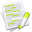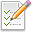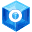#wx.Rect¶

Represents a rectangle with integer coordinates.

x and y members specify the coordinates of the rectangle top-left corner and width and height specify its width and height respectively. The usual C++ semi-open interval convention is used: point p lies inside the rectangle if and only if both conditions below are satisfied:

x <= p.x < (rect.x + rect.width)
y <= p.y < (rect.y + rect.height)


In other words, the rectangle left and right boundaries are at x and x+width-1 and its top and bottom boundaries are at y and y+height-1 respectively.

Note that the x and y coordinates may be negative, but width and height are always strictly positive for non-empty rectangles.

##Class Hierarchy¶Inheritance diagram for class Rect:

##Methods Summary¶

 __init__ Default constructor. CenterIn Returns the rectangle having the same size as this one but centered relatively to the given rectangle r. CentreIn Returns the rectangle having the same size as this one but centered relatively to the given rectangle r. Contains Returns True if the given point is inside the rectangle (or on its boundary) and False otherwise. Deflate Decrease the rectangle size. Get Return the rectangle’s properties as a tuple. GetBottom Gets the bottom point of the rectangle. GetBottomLeft Gets the position of the bottom left corner. GetBottomRight Gets the position of the bottom right corner. GetHeight Gets the height member. GetIM Returns an immutable representation of the wx.Rect object, based on namedtuple. GetLeft Gets the left point of the rectangle (the same as GetX ). GetPosition Gets the position. GetRight Gets the right point of the rectangle. GetSize Gets the size. GetTop Gets the top point of the rectangle (the same as GetY ). GetTopLeft Gets the position of the top left corner of the rectangle, same as GetPosition . GetTopRight Gets the position of the top right corner. GetWidth Gets the width member. GetX Gets the x member. GetY Gets the y member. Inflate Increases the size of the rectangle. Intersect Modifies this rectangle to contain the overlapping portion of this rectangle and the one passed in as parameter. Intersects Returns True if this rectangle has a non-empty intersection with the rectangle rect and False otherwise. IsEmpty Returns True if this rectangle has a width or height less than or equal to 0 and False otherwise. Offset Moves the rectangle by the specified offset. SetBottom Set the bottom edge of the rectangle. SetBottomLeft Set the bottom-left point of the rectangle. SetBottomRight Set the bottom-right point of the rectangle. SetHeight Sets the height. SetLeft Set the left side of the rectangle. SetPosition Sets the position. SetRight Set the right side of the rectangle. SetSize Sets the size. SetTop Set the top edge of the rectangle. SetTopLeft Set the top-left point of the rectangle. SetTopRight Set the top-right point of the rectangle. SetWidth Sets the width. SetX Sets the x position. SetY Sets the y position. Union Modifies the rectangle to contain the bounding box of this rectangle and the one passed in as parameter. __bool__ __eq__ __getitem__ __len__ __ne__ __nonzero__ __reduce__ __repr__ __setitem__ __str__ __imul__ Returns the intersection of two rectangles (which may be empty). __iadd__ Like Union , but doesn’t treat empty rectangles specially.

##Properties Summary¶

 Bottom BottomLeft BottomRight Height Left Position Right Size Top TopLeft TopRight Width X Y bottom bottomLeft bottomRight left right top topLeft topRight height A public C++ attribute of type int. Height member. width A public C++ attribute of type int. Width member. x A public C++ attribute of type int. x coordinate of the top-left corner of the rectangle. y A public C++ attribute of type int. y coordinate of the top-left corner of the rectangle.

##Class API¶

class wx.Rect(object)

Possible constructors:

Rect()

Rect(x, y, width, height)

Rect(pos, size)

Rect(size)

Rect(topLeft, bottomRight)


Represents a rectangle with integer coordinates.

### Methods¶

__init__(self, *args, **kw)Overloaded Implementations:

__init__ (self)

Default constructor.

Initializes to zero the internal x, y, width and height members.

__init__ (self, x, y, width, height)

Creates a wx.Rect object from x, y, width and height values.

Parameters
• x (int) –

• y (int) –

• width (int) –

• height (int) –

__init__ (self, pos, size)

Creates a wx.Rect object from position pos and size values.

Parameters

__init__ (self, size)

Creates a wx.Rect object from size values at the origin.

Parameters

size (wx.Size) –

__init__ (self, topLeft, bottomRight)

Creates a wx.Rect object from top-left and bottom-right points.

The width of the rectangle will be bottomRight.x-topLeft.x+1 and the height will be bottomRight.y-topLeft.y+1 .

Parameters

CenterIn(self, r, dir=BOTH)

Returns the rectangle having the same size as this one but centered relatively to the given rectangle r.

By default, rectangle is centred in both directions but if dir includes only VERTICAL or only HORIZONTAL , then it is only centered in this direction while the other component of its position remains unchanged.

Parameters
Return type

wx.Rect

CentreIn(self, r, dir=BOTH)

Returns the rectangle having the same size as this one but centered relatively to the given rectangle r.

By default, rectangle is centred in both directions but if dir includes only VERTICAL or only HORIZONTAL , then it is only centered in this direction while the other component of its position remains unchanged.

Parameters
Return type

wx.Rect

Contains(self, *args, **kw)Overloaded Implementations:

Contains (self, x, y)

Returns True if the given point is inside the rectangle (or on its boundary) and False otherwise.

Parameters
• x (int) –

• y (int) –

Return type

bool

Contains (self, pt)

Returns True if the given point is inside the rectangle (or on its boundary) and False otherwise.

Parameters

pt (wx.Point) –

Return type

bool

Contains (self, rect)

Returns True if the given rectangle is completely inside this rectangle (or touches its boundary) and False otherwise.

Parameters

rect (wx.Rect) –

Return type

bool

Deflate(self, *args, **kw)

Decrease the rectangle size.

This method is the opposite from Inflate : Deflate(a, b) is equivalent to Inflate(-a, -b). Please refer to Inflate for full description.Overloaded Implementations:

Deflate (self, dx, dy)

Parameters
• dx (int) –

• dy (int) –

Return type

wx.Rect

Deflate (self, diff)

Parameters

diff (wx.Size) –

Return type

wx.Rect

Deflate (self, diff)

Parameters

diff (int) –

Return type

wx.Rect

Get(self)

Return the rectangle’s properties as a tuple.

Return type

tuple

Returns

( x, y, width, height )

GetBottom(self)

Gets the bottom point of the rectangle.

Return type

int

GetBottomLeft(self)

Gets the position of the bottom left corner.

Return type

wx.Point

GetBottomRight(self)

Gets the position of the bottom right corner.

Return type

wx.Point

GetHeight(self)

Gets the height member.

Return type

int

GetIM(self)

Returns an immutable representation of the wx.Rect object, based on namedtuple.

This new object is hashable and can be used as a dictionary key, be added to sets, etc. It can be converted back into a real wx.Rect with a simple statement like this: obj = wx.Rect(imObj).

GetLeft(self)

Gets the left point of the rectangle (the same as GetX ).

Return type

int

GetPosition(self)

Gets the position.

Return type

wx.Point

GetRight(self)

Gets the right point of the rectangle.

Return type

int

GetSize(self)

Gets the size.

Return type

wx.Size

GetTop(self)

Gets the top point of the rectangle (the same as GetY ).

Return type

int

GetTopLeft(self)

Gets the position of the top left corner of the rectangle, same as GetPosition .

Return type

wx.Point

GetTopRight(self)

Gets the position of the top right corner.

Return type

wx.Point

GetWidth(self)

Gets the width member.

Return type

int

GetX(self)

Gets the x member.

Return type

int

GetY(self)

Gets the y member.

Return type

int

Inflate(self, *args, **kw)

Increases the size of the rectangle.

The left border is moved farther left and the right border is moved farther right by dx. The upper border is moved farther up and the bottom border is moved farther down by dy. (Note that the width and height of the rectangle thus change by 2dx and 2dy, respectively.) If one or both of dx and dy are negative, the opposite happens: the rectangle size decreases in the respective direction.

Inflating and deflating behaves “naturally”. Defined more precisely, that means:

• “Real” inflates (that is, dx and/or dy = 0) are not constrained. Thus inflating a rectangle can cause its upper left corner to move into the negative numbers. (2.5.4 and older forced the top left coordinate to not fall below (0, 0), which implied a forced move of the rectangle.)

• Deflates are clamped to not reduce the width or height of the rectangle below zero. In such cases, the top-left corner is nonetheless handled properly. For example, a rectangle at (10, 10) with size (20, 40) that is inflated by (-15, -15) will become located at (20, 25) at size (0, 10). Finally, observe that the width and height are treated independently. In the above example, the width is reduced by 20, whereas the height is reduced by the full 30 (rather than also stopping at 20, when the width reached zero).Overloaded Implementations:

Inflate (self, dx, dy)

Parameters
• dx (int) –

• dy (int) –

Return type

wx.Rect

Inflate (self, diff)

Parameters

diff (wx.Size) –

Return type

wx.Rect

Inflate (self, diff)

Parameters

diff (int) –

Return type

wx.Rect

Intersect(self, rect)

Modifies this rectangle to contain the overlapping portion of this rectangle and the one passed in as parameter.

Parameters

rect (wx.Rect) –

Return type

wx.Rect

Returns

This rectangle, modified.

Intersects(self, rect)

Returns True if this rectangle has a non-empty intersection with the rectangle rect and False otherwise.

Parameters

rect (wx.Rect) –

Return type

bool

IsEmpty(self)

Returns True if this rectangle has a width or height less than or equal to 0 and False otherwise.

Return type

bool

Offset(self, *args, **kw)

Moves the rectangle by the specified offset.

If dx is positive, the rectangle is moved to the right, if dy is positive, it is moved to the bottom, otherwise it is moved to the left or top respectively.Overloaded Implementations:

Offset (self, dx, dy)

Parameters
• dx (int) –

• dy (int) –

Offset (self, pt)

Parameters

pt (wx.Point) –

SetBottom(self, bottom)

Set the bottom edge of the rectangle.

Notice that this doesn’t affect GetTop return value but changes the rectangle height to set its bottom side to the given position.

Parameters

bottom (int) –

SetBottomLeft(self, p)

Set the bottom-left point of the rectangle.

Parameters

p (wx.Point) –

SetBottomRight(self, p)

Set the bottom-right point of the rectangle.

Parameters

p (wx.Point) –

SetHeight(self, height)

Sets the height.

Parameters

height (int) –

SetLeft(self, left)

Set the left side of the rectangle.

Notice that because the rectangle stores its left side and width, calling SetLeft changes the right side position too – but does preserve the width.

Parameters

left (int) –

SetPosition(self, pos)

Sets the position.

Parameters

pos (wx.Point) –

SetRight(self, right)

Set the right side of the rectangle.

Notice that this doesn’t affect GetLeft return value but changes the rectangle width to set its right side to the given position.

Parameters

right (int) –

SetSize(self, s)

Sets the size.

Parameters

s (wx.Size) –

SetTop(self, top)

Set the top edge of the rectangle.

Notice that because the rectangle stores its top side and height, calling SetTop changes the bottom side position too – but does preserve the height.

Parameters

top (int) –

SetTopLeft(self, p)

Set the top-left point of the rectangle.

Parameters

p (wx.Point) –

SetTopRight(self, p)

Set the top-right point of the rectangle.

Parameters

p (wx.Point) –

SetWidth(self, width)

Sets the width.

Parameters

width (int) –

SetX(self, x)

Sets the x position.

Parameters

x (int) –

SetY(self, y)

Sets the y position.

Parameters

y (int) –

Union(self, rect)

Modifies the rectangle to contain the bounding box of this rectangle and the one passed in as parameter.

Parameters

rect (wx.Rect) –

Return type

wx.Rect

__bool__(self)

__eq__(self, other)
Return type

bool

__getitem__(self, idx)

__len__(self)

__ne__(self, other)
Return type

bool

__nonzero__(self)

__reduce__(self)

__repr__(self)

__setitem__(self, idx, val)

__str__(self)

__imul__(self)

Returns the intersection of two rectangles (which may be empty).

Parameters

r (wx.Rect) –

__iadd__(self)

Like Union , but doesn’t treat empty rectangles specially.

Parameters

r (wx.Rect) –

### Properties¶

Bottom

BottomLeft

BottomRight

Height

Left

Position

Right

Size

Top

TopLeft

TopRight

Width

X

Y

bottom

bottomLeft

bottomRight

height

A public C++ attribute of type int. Height member.

left

right

top

topLeft

topRight

width

A public C++ attribute of type int. Width member.

x

A public C++ attribute of type int. x coordinate of the top-left corner of the rectangle.

y

A public C++ attribute of type int. y coordinate of the top-left corner of the rectangle.